### Demand Theory and General Equilibrium: An Intermediate Level Introduction to MPSGE

### 1995

* This research supported by the GAMS Applied General Equilibrium Research Fund. The software described here operates only with GAMS 2.25.085 or later on the PC, shipped in July, 1995. The author remains responsible for any bugs which exist in this software.

### 1. An Overview

This document describes a mathematical programming system for general equilibrium analysis named MPSGE which operates as a subsystem to the mathematical programming language GAMS. MPSGEis library of function and Jacobian evaluation routines which facilitates the formulation and analysis of AGE models. MPSGE simplifies the modeling process and makes AGE modeling accessible to any economist who is interested in the application of these models. In addition to solving specific modeling problems, the system serves a didactic role as a structured framework in which to think about general equilibrium systems.

MPSGE separates the tasks of model formulation and model solution, thereby freeing model builders from the tedious task of writing model-specific function evaluation subroutines. All features of a particular model are communicated to GAMS/MPSGE through a tabular input format. To use MPSGE, a user must learn the syntax and conventions of this model definition language.

The present paper is intended for students who have completed two semesters of study in microeconomics. The purpose of this presentation is to give students a practical perspective on microeconomic theory. The diligent student who works through all of the examples provided here should be capable of building small models "from scratch" to illustrate basic theory. This is a first step to acquiring a set of useable tools for applied work.

The remainder of this paper is organized as follows. Section 2 review the theory of consumer demand; section 3 provides guidance on the mechanics of numerical modeling, including instructions on how to install and test the GAMS/MPSGE software; section 4 introduces the modeling framework with three models illustrating the representation of consumer demand within the MPSGE language. Section 5 reviews the pure exchange model, and section 6 presents two MPSGE models of exchange. Each of the model- oriented sections present exercises based on the models which give students a chance to work through the material on their own. Additional introductory examples for self-study can be found in the Markusen library , as well as in the GAMS model library (look for models with names ending in "MGE").

The level of presentation and diagrammatic exposition adopted here is based on Hal Varian's undergraduate microeconomics textbook ( Intermediate Microeconomics: A Modern Approach, Third Edition, W. W. Norton & Company, Inc., 1993).

The ultimate objective of this piece is to remind students of some theory which they have already seen and illustrate how these ideas can be used to build numerical models using GAMS with MPSGE. It is not my intention to provide a graduate level presentation of this material. So far as possible, I have avoided calculus and even algebra. The objective here is to demonstrate that what matters are economic ideas. With the proper tools, it is possible to do concrete economic modeling without a lot of mathematical formalism.

### 2. The Theory of Consumer Demand

A central idea underlying most microeconomic theory is that agents optimize subject to constraints. The optimizing principle applied to consumer choice begins from the notion that agents have preferences over consumption bundles and will always choose the most preferred bundle subject to applicable constraints. To operationalize this theory, three issues which must be addressed: (i) How can we represent preferences? (ii) What is the nature of constraints on consumer choice? and (iii) How can the choice be modelled?

Preferences are relationships between alternative consumption "bundles". These can be represented graphically using "indifference curves", as illustrated in Figure 1. Focusing now on the preferences of a single consumer, the indifference curve is a line which connects all combinations of two goods x and y between which our consumer is indifferent. As this curve is drawn, we have represented an agent with "well-behaved preferences": at any allocation, more is better (monotonicity), and averages are preferred to extremes (convexity). Exactly one such indifference curve goes through each positive combination of x and y. Higher indifference curves lie to the "north-east".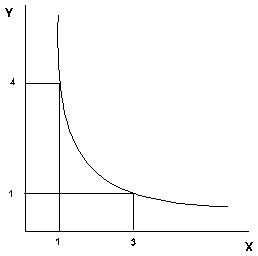#### Figure 1: An Indifference Curve

If we wish to characterize an agent's preferences, the "marginal rate of substitution" (MRS) is a useful point of reference. At a given combination of x and y, the marginal rate of substitution is the slope of the associated indifference curve. As drawn, the MRS increases in magnitude as we move to the northwest and the MRS decreases as we move to the south east. The intuitive understanding is that the MRS measures the willingness of the consumer to trade off one good for the other. As the consumer has greater amounts of x, she will be willing to trade more units of x for each additional unit of y -- this results from convexity.

An "ordinal" utility function U(x,y) provides a helpful tool for representing preferences. This is a function which associates a number with each indifference curve. These numbers increase as we move to the northeast, with each successive indifference curve representing bundles which are preferred over the last. The particular number assigned to an indifference curve has no intrinsic meaning. All we know is that if U(x1,y1) > U(x2,y2) , then the consumer prefers bundle 1 to bundle 2.

Figure 2 illustrates how it is possible to use a utility function to generate a diagram with the associated indifference curves. This figure illustrates Cobb-Douglas well-behaved preferences which are commonly employed in applied work.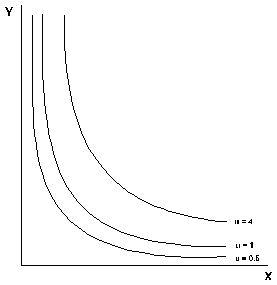#### Figure 2: Indifference Curves and Utility Levels

Up to this point, we have we have focused exclusively on the characterization of preferences. Let us now consider the other side of the consumer model -- the budget constraint. The simplest approach to characterizing consumer income is to assume that the consumer has a fixed money income which she may spend on any goods. The only constraint on this choice is that the value of the expenditure may not exceed the money income. This is the standard budget constraint:

Px x + Py y = M.

Graphically, this equation defines the line depicted in Figure 3. All points inside the budget line are affordable. The consumer faces a choice of which affordable bundle to select.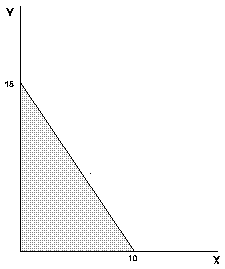#### Figure 3: The Budget Set

Within the framework of our theory, the answer is straightforward -- the consumer will choose the one combination of x and y from the set of affordable bundles which maximizes her utility. This combination of x and y will be at the point where the indifference curve is tangent the budget line. This point is called the optimal choice. We see this illustrated in Figure 4.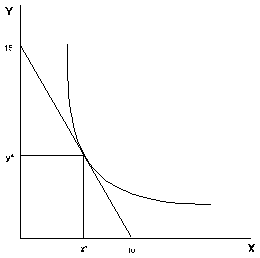#### Figure 4: Utility Maximization on the Budget Set

The standard model of consumer behavior provides a starting point for learning MPSGE. This introduction is "hands on" -- I will discuss issues as they arise, assuming that you have access to a computer and can invoke the program and read the output file. You may wish to learn the rudiments of GAMS syntax before starting out, although you may be able to pick up these ideas as they come, depending on your aptitude for computer languages.

### 3. Getting Started with GAMS/MPSGE

(i) You need to know how to generate a standard text file. There are several methods for doing this. One approach is to use EDIT, the standard DOS text editor. Another approach favored by many people who have used text processors like WordPerfect or Microsoft Word is to use the word processor as a text editor. A disadvantage of this approach is that you will need to remember to always save the edited file in a text format. A final approach, one which I suggest to graduate students who are interested in numerical modeling, is that you develop facility with a "real" programmer's editor like Emacs, Epsilon or Brief. These editors are far more powerful than the DOS Edit, and they are far better suited to computational work than a word processor.

(ii) You need to have a copy of GAMS with MPSGE to run on your computer. There are versions of this program for 386/486/586 PCs as well as for most widely-used workstations (SUN,DEC,HP,IBM,etc.). Copies of the program can be obtained directly from GAMS (gams@gams.com), or you may take a copy from someone who already has the program. (Copyright restrictions apply only to GAMS license files.) You may copy the GAMS programs without the license files and the program will only operate in student/demonstration mode. The student version is perfectly adequate for learning about modeling --in fact, it may be better because it's dimensionality restrictions prevent the novice model builder from adding unnecessary details.

(iii) You need to install GAMS with MPSGE on your computer. To do this, follow the standard installation procedure:

(a) Make a GAMS system directory, e.g.

```        c:\>mkdir gams
```

(b) Copy all the files from the GAMS distribution diskette(s) into the GAMS system directory; e.g.

```        c:\>copy a:*.* c:\gams
```

(c) Connect to the GAMS system directory and run the GAMS installation program e.g.

```      c:\>cd gams
c:\gams>gamsinst
```

The installation program gives you choices regarding which solver you wish to use for various problem classes. For MPSGE models, the key choice is the MCP solver. I recommend that you select PATH if you have this solver on your computer. It is the most efficient and robust solver currently available for this class of models. (PATH by Michael Ferris and Steve Dirkse of the University of Wisconsin at Madison.)

You complete the installation by adding the GAMS system directory to your DOS environment variable, PATH. Normally, PATH is initialized at startup in the file C:\>AUTOEXEC.BAT. Look for a line which looks like "`PATH C:\DOS`" and modify this to read something like: "`PATH C:\DOS;C:\GAMS`" using a text editor.

(iv) You should verify that the system is operational. Connect to a working directory (never run models from the GAMS system directory!). Then, extract and run one of the library models, e.g.

```      C:\>MKDIR WORK
C:\>CD WORK
C:\WORK>GAMSLIB SCARFMGE
C:\WORK>GAMS SCARFMGE
```

If the GAMS system is properly installed, these commands will cause GAMS to solve a sequence of models from the ` SCARFMGE ` sample problem. The output from this process is written to file ` SCARFMGE.LST `. If you search for the word "STATUS", you can verify that all the cases are processed.

There are a number of MPSGE models included in the GAMS library. If you are using a student version of GAMS, you will be able to process some but not all of the library models. The student version of the program limits the number of variables in the model to 100. (I believe that GAMS imposes other limits on use of the student version, but the variable limitation is the most severe constraint.)

Assuming that you have successfully installed the software, let us now proceed to some examples which illustrate both the computing syntax and the underlying economics.

### 4. Modeling Consumer Demand with MPSGE

#### Example 1 : Evaluating a Demand Function

Consider a standard consumer choice problem, one which might appear on a midterm examination in intermediate microeconomics:

max U(x,y) = ln(x) + 2 ln(y)

subject to:
1 x + 2 y = 120

where 1 is the exogenous price of x and 2 is the price of y.

This type of problem is solved easily using GAMS/MINOS (as a nonlinear program). Strictly speaking, it is not the sort of model for which you would need to use MPSGE. At the same time, this can be an instructive example.

The key issue in this example is learning how to represent utility functions. MPSGE is "non-algebraic" -- so function specification depends on an intuitive understanding of the underlying economic structure.

Consider Figure 5 and focus on a single point, x=1, y=1. There is an indifference curve through this point, and the marginal rate of substitution (MRS) at this point is simply the slope of this curve. The benchmark MRS does not uniquely determine the underlying preferences.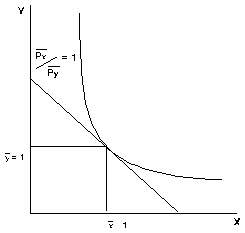#### Figure 5: A Calibrated Benchmark

A utility function is represented in MPSGE by the specification of: (i) benchmark demand quantities, (ii) benchmark demand prices (iii) an elasticity of substitution at the benchmark point. Benchmark quantities determine an anchor point for the set of indifference curves. Benchmark prices fix the slope of the indifference curve at that point, and the elasticity describes the curvature of the indifference curve. Speaking formally, elasticities provide a "second order approximation" of the utility function. To understand the importance of the benchmark elasticity of substitution, consider Figure 6. This figure shows three indifference curves all of which share the same benchmark quantities and benchmark prices. They differ only in the elasticities of substitution. The least convex (flattest) curve has the highest elasticity, the most convex curve has the lowest elasticity. (When the elasticity of substitution is 0, the indifference curve is L-shaped with the corner at the benchmark point.)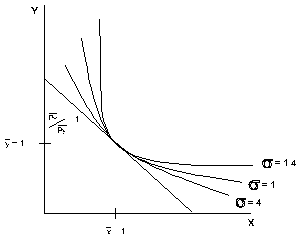#### Figure 6: The Elasticity of Substitution

Let us now consider how the consumer optimization problem can be cast as a general equilibrium model. We do this by adding a single factor of production and two "production" sectors. For concreteness, let the factor of production be called labor with a price PL. One production function converts one unit of labor into one unit of x, the other sector converts 2 units of labor into one unit of y. Setting the labor endowment equal 120, the market clearance condition for labor reads:

1 x + 2 y = 120

which is precisely the budget constraint for the consumer's problem.

We will now present the program code, a few lines at a time. As part of working through the example, the student should type these lines into a file.

A MPSGE model specification is always listed between ` \$ONTEXT ` and `\$OFFTEXT` statements. The first statement within an MPSGE model-description assigns a name to the model. The model name must begin with a letter and must have 10 or fewer characters.

```\$ONTEXT

\$MODEL:DEMAND
```

The model specification begins by declaring variables for the model. In a standard model, there are three types of variables: commodity prices, sectoral activity levels, and consumer incomes. The end of each line may include "! variable description ".

N.B. The variables associated with commodities are prices, not quantities. (In this and subsequent models, I use P as the first letter for each of the commodity variables to remind us that these variables are prices.)

N.B. The variable associated with a consumer is an income level, not a welfare index.

```\$SECTORS:
X       ! ACTIVITY LEVEL FOR X = DEMAND FOR GOOD X
Y       ! ACTIVITY LEVEL FOR Y = DEMAND FOR GOOD Y

\$COMMODITIES:
PX      ! PRICE OF X WHICH WILL EQUAL PL
PY      ! PRICE OF Y WHICH WILL EQUAL 2 PL
PL      ! PRICE OF THE ARTIFICIAL FACTOR L

\$CONSUMERS:
RA      ! REPRESENTATIVE AGENT INCOME
```
Function specifications follow the variable declarations. In this model, our first declarations correspond to the two production sectors. In this model, the production structures are particularly simple. Each of the sectors has one input and one output. In the MPSGE syntax, `I:` denotes an input and `O:` denotes an output. The output quantity coefficients for both sectors are unity (`Q:1`). This means that the level values for x and y correspond to the actual quantities produced.

The final function specified in the model represents the utility function and endowments for our single consumer. In this function, the `E:` entries correspond to endowments and the `D:` entries are demands. Reference demands, reference prices and the substitution elasticity (`s:1`) characterize preferences.

The demand entries shown here are consistent with a Cobb-Douglas utility function in which the budget share for y is twice the budget share for x (i.e. the MRS at (1,1) equals 1/2):

```\$PROD:X
O:PX  Q:1
I:PL  Q:1

\$PROD:Y
O:PY  Q:1
I:PL  Q:2

\$DEMAND:RA  s:1
E:PL  Q:120
D:PX  Q:1   P:(1/2)
D:PY  Q:1   P:1

\$OFFTEXT
```

The final three statements in this file invoke the MPSGE preprocessor, "generate" and solve the model:

```\$SYSINCLUDE mpsgeset DEMAND

\$INCLUDE DEMAND.GEN
SOLVE DEMAND USING MCP;
```

The preprocessor invocation (\$SYSINCLUDE mpsgeset") should be placed immediately following the `\$OFFTEXT` block containing the model description. The model generator code, `DEMAND.GEN`, is produced by the previous statement and must be referenced immediately before each subsequent `SOLVE` statement.

At this point, the reader should take the time to type the example into a file and execute the program with GAMS/MPSGE.

This is possibly the first GAMS model which some readers have solved, so it is worth looking through the listing file in some detail. After running the solver, we examine the listing file. I typically begin my assessment of a model's solution by searching for "STATUS". For this model, we have the following:

```               S O L V E      S U M M A R Y

MODEL   DEMAND
TYPE    MCP
SOLVER  PATH                FROM LINE  263

**** SOLVER STATUS     1 NORMAL COMPLETION
**** MODEL STATUS      1 OPTIMAL

RESOURCE USAGE, LIMIT          1.432     1000.000
ITERATION COUNT, LIMIT         5         1000
EVALUATION ERRORS              0            0

Work space allocated           --    4.86 Mb

Default price normalization using income for RA
```

This information is largely self-explanatory. The most important items are the SOLVER STATUS and MODEL STATUS indicators. When the solver status is 1 and the model status is 1, the system has returned an equilibrium.

For small models such as this, the limits on resource usage (time) and solver iterations have no effect. (You can modify these values with the statements:

``` model.RESLIM  =  number of cpu seconds ;
model.ITERLIM =  number of iterations ;
```
entered into the program before the `SOLVE` statement.)

The work space allocation for MPSGE models is determined by the number of variables in the model. It is possible to exogenously specify the work space allocation by assigning

``` model.WORKSPACE = xx ; ```

where ` xx ` is the desired number of megabytes.

The final message, "Default price normalization...", is significant. It reminds the user that an Arrow-Debreu general equilibrium model determines only relative prices. In such an equilibrium, the absolute scaling of prices is indeterminant. (I.e., if (p*,M*) are a set of equilibrium prices and income levels, then (2 p*,2 M*) is also a solution, etc.)

It is common practice in economics to address the normalization issue through the specification of a numeraire commodity. You can do this for an MPSGE model by "fixing" a price, with a statement like:

``` PX.FX = 1; ```

entered following the model declaration (\$SYSINCLUDE mpsgeset) but prior to the solver invocation. When any price or income level is fixed, MPSGE recognizes that a numeraire has been specified and does no automatic normalization.

Following some output from the solver (PATH in this case), the listing file provides a complete report of equilibrium values. With MPSGE models, the equation listings are superfluous. The variable listings provide all the relevant information.

For this model, the solution listing appears as follows:

```                       LOWER     LEVEL     UPPER    MARGINAL
---- VAR X               .       40.000     +INF       .
---- VAR Y               .       40.000     +INF       .
---- VAR PX              .        1.000     +INF       .
---- VAR PY              .        2.000     +INF       .
---- VAR PL              .        1.000     +INF       .
---- VAR RA              .      120.000     +INF       .

X           SUPPLY AND DEMAND OF GOOD X
Y           SUPPLY AND DEMAND OF GOOD Y
PX          PRICE OF X WHICH WILL EQUAL CX * PL
PY          PRICE OF Y WHICH WILL EQUAL CY * PL
PL          PRICE OF THE ARTIFICIAL FACTOR L
RA          REPRESENTATIVE AGENT INCOME
```
The LOWER and UPPER columns report variable bounds applied in the model. In these columns, zero is represented by "." and infinity is represented by `"+INF"`. The LEVEL column reports the solution value returned by the algorithm. Here we see that the equilibrium price of x is 1 and the price of y is 2, as determined by the specification of labor inputs.

#### Exercises based on example 1:

(a) The utility function calibration point is arbitrary. Here, we have selected x=y=1 as the reference quantity. Revise the program to use a different calibration point where x=2 and y=1, where MRS(2,1) = 1/4. (Remember to modify both the `Q:` and `P:` fields.) Rerun the model to demonstrate that this does not change the result.

(b) Increase the price of x from 1 to 2 by changing the Q: coefficient for PL in sector X from 1 to 2. What happens to the demand for x? Explain why a change in the price of x is represented by a change in the Q: field for sector X.

(c) Compute an equilibrium in which commodity y is defined as the numeraire.

#### Example 2 : Evaluating the Marginal Rate of Substitution

This example further explores the representation of demand functions with MPSGE. It sets up a trivial equilibrium model with two goods and one consumer which returns the marginal rate of substitution of good x for good y at a given level of demand.

The underlying utility function is:

U(x,y) = ln(x) + 4 ln(y)

When x=y=1, the marginal rate of substitution of x for y is 1/4. We use this information to calibrate the demand function, specifying the ratio of the reference prices of x to y equal to 1/4.

In an equilibrium, final demand always equals endowments for both goods, because these are the only sources of demand and supply. The model as parameterized demonstrates that if we set endowments for this model equal to the demand function calibration point, the model equilibrium price ratio equals the benchmark MRS.

This program begins with some GAMS statements in which three scalar parameters are declared. These parameters will be used in the place of numbers within the MPSGE model. The syntax for these GAMS statements is introduced in Chapter 2 of the GAMS manual. In short, we declare x, y and MRS as scalar parameters and initialize the first two of these to unity. The MRS parameter is assigned a value following the solution of the model.

```
SCALAR
X     QUANTITY OF X FOR WHICH THE MRS IS TO BE EVALUATED /1/
Y     QUANTITY OF Y FOR WHICH THE MRS IS TO BE EVALUATED /1/
MRS   COMPUTED MARGINAL RATE OF SUBSTITUTION;
```

The remainder of the MPSGE program is, in fact, simpler than Example 1.

```\$ONTEXT

\$MODEL:MRSCAL

\$COMMODITIES:
PX    ! PRICE INDEX FOR GOOD X
PY    ! PRICE INDEX FOR GOOD Y

\$CONSUMERS:
RA    ! REPRESENTATIVE AGENT

\$DEMAND:RA  s:1
D:PX  Q:1   P:(1/4)
D:PY  Q:1   P:1
E:PX  Q:X
E:PY  Q:Y
\$OFFTEXT
\$SYSINCLUDE mpsgeset MRSCAL

\$INCLUDE MRSCAL.GEN
SOLVE MRSCAL USING MCP;
```

Following the solution, we compute a function of the solution values, the ratio of the price of x to the price of y. We do this using the GAMS syntax which references the equilibrium level values of the `PX` and ```PY ```and storing this result in the scalar MRS. This scalar value is then displayed in the listing file with 8 digits:

```MRS = PX.L / PY.L;
OPTION MRS:8;
DISPLAY MRS;
```

#### Exercises for the second example problem:

(a) Show that the demand function is homothetic by uniform scaling of the x and y endowments. The resulting MRS should remain unchanged.

(b) Modify the demand function calibration point so that the reference prices of both x and y equal unity (hint: the marginal rate of substitution is:

MRS = x / (4 y).

#### Example 3 : Goods Demand, Leisure Demand and Labor Supply

This model investigates the labor-leisure decision. A single consumer is endowed with labor which is either supplied to the market or "repurchased" as leisure. The consumer utility function over market goods (x and y) and leisure is Cobb-Douglas:

U(x,y,L) = ln(x) + ln(y) + ln(L)

Goods x and y may only be purchased using funds obtained from labor sales. This constraint is written:

x + y = LPROD LS

where goods x and y both have a price of unity at base year productivity and LPROD is an index of labor productivity. An increase in productivity is equivalent to a proportional decrease in the prices of x and y.

The model declaration is as follows:

```SCALAR          LPROD   AGGREGATE LABOR PRODUCTIVITY /1/,
CX      COST OF X AT BASE YEAR PRODUCTIVITY /1/,
CY      COST OF Y AT BASE YEAR PRODUCTIVITY /1/;
\$ONTEXT

\$MODEL:LSUPPLY

\$SECTORS:
X       ! SUPPLY=DEMAND FOR X
Y       ! SUPPLY=DEMAND FOR Y
LS      ! LABOR SUPPLY

\$COMMODITIES:
PX      ! MARKET PRICE OF GOOD X
PY      ! MARKET PRICE OF GOOD Y
PL      ! MARKET WAGE
PLS     ! CONSUMER VALUE OF LEISURE

\$CONSUMERS:
RA      ! REPRESENTATIVE AGENT

\$PROD:LS
O:PL    Q:LPROD
I:PLS   Q:1

\$PROD:X
O:PX    Q:1
I:PL    Q:CX

\$PROD:Y
O:PY    Q:1
I:PL    Q:CY

\$DEMAND:RA  s:1
E:PLS   Q:120
D:PLS   Q:1     P:1
D:PX    Q:1     P:1
D:PY    Q:1     P:1

\$OFFTEXT
\$SYSINCLUDE mpsgeset LSUPPLY

\$INCLUDE LSUPPLY.GEN
SOLVE LSUPPLY USING MCP;
```
We can use this model to evaluate the wage elasticity of labor supply. In the initial equilibrium (computed in the last statement) the demands for x, y and L all equal 40. A subsequent assignment to LPROD (below) increases labor productivity. After computing a new equilibrium, we can use the change in labor supply to determine the wage elasticity of labor supply, an important parameter in labor market studies.

It should be emphasized that the elasticity of labor supply should be an input rather than an output of a general equilibrium model -- this is a parameter for which econometric estimates can be obtained.

Here is how the programming works. First, we declare some scalar parameters which we will use for reporting, then save the "benchmark" labor supply in LS0:

```SCALAR
LS0   REFERENCE LEVEL OF LABOR SUPPLY
ELS   ELASTICITY OF LABOR SUPPLY WRT REAL WAGE;

LS0 = LS.L;
```
Next, we modify the value of scalar LPROD, increasing labor productivity by 1%. Because this is a neoclassical model, this change is equivalent to increasing the real wage by 1%. We need to recompute equilibrium prices after having changed the LPROD value:

```LPROD = 1.01;
\$INCLUDE LSUPPLY.GEN
SOLVE LSUPPLY USING MCP;
```
We use this solution to compute and report the elasticity of labor supply as the percentage change in the LS activity:

```ELS = 100 * (LS.L - LS0) / LS0;
DISPLAY ELS;
```
As the model is currently constructed, the wage elasticity of labor supply equals zero. This is because the utility function is Cobb-Douglas over goods and leisure, and the consumer's only source of income is labor. As the real wage rises, this increases both the demand for goods (labor supply) and the demand for leisure. These effect exactly balance out and the supply of labor is unchanged.

#### Exercises based on example 3:

(a) One way in which the labor supply elasticity might differ from zero in a model with Cobb-Douglas final demand is if there were income from some other source. Let the consumer be endowed with good x in addition to labor. What x endowment is consistent with a labor supply elasticity equal to 0.15?

(b) A second way to "calibrate" the labor supply elasticity is to change the utility function. We can do this by changing the `s:1 `to `s:SIGMA`, where `SIGMA `is a scalar value representing the benchmark elasticity of substitution between x, y and L in final demand. Modify the program to include `SIGMA `as a scalar, and find the value for `SIGMA `consistent with a labor supply elasticity equal to 0.15.

### 5. The Pure Exchange Model

Partial equilibrium analysis forms the basis of most economics courses at the undergraduate level. In these models we focus on price, supply and demand for a single commodity. The partial equilibrium approach neglects indirect effects, through which changes in the market for one good may influence the market for another good.

In the previous section, we focused on the choices of a single consumer. In the present section, we will explore the implications of interactions between many consumers with heterogeneous preferences. Furthermore, the analysis will explore the potentially important interaction between market prices and income which are determined jointly in a general equilibrium.

The most widely-used graphical framework for multi-agent exchange equilibrium analysis is the Edgeworth-Bowley box as illustrated in Figure 7. In this diagram we model the following economy: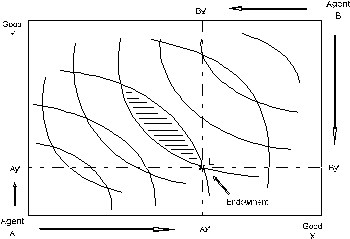#### Figure 7: The Edgeworth-Bowley Box

* Two types of consumers, denoted A and B. We consider A and B to each represent multiple consumers, each with the same endowments and preferences. This assumption is helpful for justifying our assumption of perfectly competitive, price-taking behavior.

* Two commodities, denoted x and y

* Fixed endowments of both goods. The horizontal axis measures the total world endowment of good X. The vertical axis measure the total world endowment of good Y. Any point in the box then represents an allocation of goods between the two agents. The agent H allocation is measured with respect to the lower left origin. The agent F allocation is measured with respect to the upper right origin.

Each agent has a given initial endowment, here denoted point E. Furthermore, we assume that there is no possibility for trade. The indifference curves through point E therefore represent autarchy welfare levels.

The key idea in this model is that trade can improve both agents' welfare. One agent gives some amount good x to the other in return for an amount of good y. The "terms of trade", the rate of exchange between x and y, is determined by the model. The model illustrates a number of important properties of market economies:

• Trade is mutually beneficial. So long as the transactions are voluntary, neither H nor F will be hurt by engaging in trade.

• Market prices can be used to guide the economy to a Pareto-efficient allocation, a state of affairs in which further mutually-beneficial trades are not possible.

• There is no guarantee that the gains from trade will be "fairly distributed" across consumers. A competitive equilibrium may produce a significant welfare increase for one consumer while have negligible impact on the other.

• There are multiple Pareto-efficient allocations, typically only one of which is a competitive equilibrium. We can use this model to demonstrate that the issues of efficiency and equity can be separated when there is the possibility of lump-sum income transfers between agents.

### 6. modeling Pure Exchange with MPSGE

#### Example 4: A 2x2 Exchange Model

In this program, we examine the simple two good, two agent model of exchange equilibrium. The world endowments for goods x and y are both equal to 1. Six parameters are used to parameterize the model. These are declared as scalars at the top of the program:

```SCALAR  XA              AGENT A ENDOWMENT OF X ( 0 < XA < 1)    /0.2/
YA              AGENT A ENDOWMENT OF Y ( 0 < YA < 1)    /0.8/
THETA_A         AGENT A DEMAND SHARE PARAMETER FOR X    /0.5/
THETA_B         AGENT B DEMAND SHARE PARAMETER FOR X    /0.8/
SIGMA_A         AGENT A ELASTICITY PARAMETER            /2.0/
SIGMA_B         AGENT B ELASTICITY PARAMETER            /0.5/;
```
This model is actually simpler than the models presented above because we have no need for production. There are simply two commodities and two consumers. The consumers differ in terms of commodity endowments and preferences. The competitive equilibrium prices are such that supply equals demand for both goods and both agents spend an amount equal to their endowment income.

This model illustrates how to use computed function coefficients. See, for example, `Q:(1-THETA_A)` in the `\$DEMAND:A` block. Any numeric input field in an MPSGE model may be "computed", provided that the algebraic expression is enclosed within parentheses and legitimate GAMS code.

This model specification uses the default values for reference prices in the demand function blocks. When `P:value` is not specified in a `D:`,`I:` or O: record, `P:1` is assumed.

This model uses the more general constant-elasticity-of-substitution utility function.

```\$ONTEXT

\$MODEL:EXCHANGE

\$COMMODITIES:
PX    ! EXCHANGE PRICE OF GOOD X
PY    ! EXCHANGE PRICE OF GOOD Y

\$CONSUMERS:
A     ! CONSUMER A
B     ! CONSUMER B

\$DEMAND:A  s:SIGMA_A
E:PX  Q:XA
E:PY  Q:YA
D:PX  Q:THETA_A
D:PY  Q:(1-THETA_A)

\$DEMAND:B  s:SIGMA_B
E:PX  Q:(1-XA)
E:PY  Q:(1-YA)
D:PX  Q:THETA_B
D:PY  Q:(1-THETA_B)

\$OFFTEXT
\$SYSINCLUDE mpsgeset EXCHANGE

\$INCLUDE EXCHANGE.GEN
SOLVE EXCHANGE USING MCP;

SCALAR
PRATIO  EQUILIBRIUM PRICE OF X IN TERMS OF Y,
IRATIO  EQUILIBRIUM RATIO OF CONSUMER A INCOME TO CONSUMER B INCOME;

PRATIO = PX.L / PY.L;
IRATIO = A.L  / B.L;

DISPLAY IRATIO, PRATIO;
```
The foregoing sets up the model and computes the competitive equilibrium. After GAMS returns from the solver, we declare and compute some report values.

Absolute levels of income and price returned from a general equilibrium model are not meaningful because a model determines only relative prices. For this reason, we report equilibrium income and price levels in relative terms.

In the final step, we compute an alternative efficient equilibrium, one in which the income levels for A and B are equal. The purpose of this exercise is to demonstrate the second welfare theorem. When incomes are both fixed, the equilibrium remains efficient, but the connection between market prices and endowment income is eliminated.

In GAMS/MPSGE, a variable may be fixed using the GAMS syntax

```variable.fx= value;```

as illustrated in this model:

```A.FX = 1;
B.FX = 1;
\$INCLUDE EXCHANGE.GEN
SOLVE EXCHANGE USING MCP;

SCALAR      TRANSFER  IMPLIED TRANSFER FROM A TO B AS A PERCENTAGE OF INCOME;

TRANSFER = 100 * ( A.L - PX.L * XA - PY.L * YA ); PRATIO = PX.L / PY.L;
IRATIO = A.L  / B.L;

DISPLAY TRANSFER, PRATIO, IRATIO;
```

#### Exercises for example 4:

(a) Set up a separate models which computes the autarchy price ratios for consumers A and B. (You can use one of the earlier models as a starting point.)

(b) Determine parameter values for which the endowment point is the equilibrium point.

(c) Set up a series of computations from which you can sketch the efficiency locus. Draw the Edgeworth box diagram which is consistent with these values.

#### Example 5: Import Tariffs and Market Power in an Exchange Model

The exchange model provides a remarkably useful tool for analyzing issues related to international trade. Formal trade theory is more complicated with the inclusion of separate production technologies. We will present some of those models below. Before going forward, however, we will consider a slight generalization of the 2x2 model exchange model. In this extension, we introduce independent markets for consumers A and B and trade activities which deliver goods from one market to the other.

The set of input parameters largely the same as in the previous example. Two new parameters are ad-valorem tariffs which apply on imports to each of the regions.

```SCALAR  XA              AGENT A ENDOWMENT OF X ( 0 le XA le 1)    /0.2/
YA              AGENT A ENDOWMENT OF Y ( 0 le YA le 1)    /0.8/
THETA_A         AGENT A DEMAND SHARE PARAMETER FOR X    /0.4/
THETA_B         AGENT B DEMAND SHARE PARAMETER FOR X    /0.6/
SIGMA_A         AGENT A ELASTICITY PARAMETER            /1.0/
SIGMA_B         AGENT B ELASTICITY PARAMETER            /1.0/,
T_A             AD-VALOREM TARIFF ON IMPORTS TO AGENT A /0.10/
T_B             AD-VALOREM TARIFF ON IMPORTS TO AGENT B /0.10/;
```

The program differs from the previous example in several respects. First, we introduce a separate commodity price for each agent. In the absence of tariffs, these prices are identical.

A second difference is that in this model trade activities deliver goods from one agent to the other. These are denoted M{good}{agent} for imports of {good} to {agent}. There are four flows which may be operated in only one direction (the activity levels are non-negative). In terms of initial endowments and preferences, this model has exactly the same economic structure as the previous model.

```\$ONTEXT

\$MODEL:TARIFFS

\$SECTORS:
MXA     ! TRADE IN X FROM B TO A
MXB     ! TRADE IN X FROM A TO B
MYA     ! TRADE IN Y FROM B TO A
MYB     ! TRADE IN Y FROM A TO B

\$COMMODITIES:
PXA     ! PRICE OF GOOD X FOR AGENT A
PYA     ! PRICE OF GOOD Y FOR AGENT A
PXB     ! PRICE OF GOOD X FOR AGENT B
PYB     ! PRICE OF GOOD Y FOR AGENT B

\$CONSUMERS:
A       ! CONSUMER A
B       ! CONSUMER B

\$DEMAND:A  s:SIGMA_A
E:PXA   Q:XA
E:PYA   Q:YA
D:PXA   Q:THETA_A
D:PYA   Q:(1-THETA_A)

\$DEMAND:B  s:SIGMA_B
E:PXB   Q:(1-XA)
E:PYB   Q:(1-YA)
D:PXB   Q:THETA_B
D:PYB   Q:(1-THETA_B)
```

The trade activities each have one input and one output. They simply deliver a good (X or Y) from one agent to the other. The new syntax presented here is specification of an ad-valorem tax. Adding a tax requires two new fields. The first is "A:" which specifies the tax agent, a consumer who collects the tax revenue as part of income. The second is "T:" which specifies the ad- valorem tax rate.

MPSGE permits taxes to applied on production inputs and outputs but it does not permit taxes on final demand.

The tax applies on a net basis on inputs. For example, if we consider the MXA sector, the price of one unit of input is given by:

Px_B * (1 + Ta)

where Px_B is the net of tax price of a unit of x in the agent B market and Ta is the ad-valorem tariff rate.

```\$PROD:MXA
O:PXA Q:1
I:PXB Q:1   A:A  T:T_A

\$PROD:MXB
O:PXB Q:1
I:PXA Q:1   A:B  T:T_B

\$PROD:MYA
O:PYA Q:1
I:PYB Q:1   A:A  T:T_A

\$PROD:MYB
O:PYB Q:1
I:PYA Q:1   A:B  T:T_B
```

The final portions of the file introduces one use of "MPSGE report variables". In this case, report variables are used to recover a Hicksian money-metric welfare index for each of the agents. We compute the initial, tariff-ridden equilibrium in order to compute the benchmark welfare levels. We then set all tariffs to zero and compute the free-trade equilibrium. Using the final welfare indices and the saved values of the benchmark welfare levels, we are able to report the change in welfare from removing tariff distortions.

```\$REPORT:
V:WA  W:A
V:WB  W:B

\$OFFTEXT
\$SYSINCLUDE mpsgeset TARIFFS

\$INCLUDE TARIFFS.GEN
SOLVE TARIFFS USING MCP;

SCALAR
WA0   BENCHMARK WELFARE INDEX FOR AGENT A
WB0   BENCHMARK WELFARE INDEX FOR AGENT B;

WA0 = WA.L;
WB0 = WB.L;
T_A = 0;
T_B = 0;

\$INCLUDE TARIFFS.GEN
SOLVE TARIFFS USING MCP;

SCALAR
EVA   HICKSIAN EQUIVALENT VARIATION FOR AGENT A
EVB   HICKSIAN EQUIVALENT VARIATION FOR AGENT B;

EVA = 100 * (WA.L-WA0)/WA0;
EVB = 100 * (WB.L-WB0)/WB0;
DISPLAY EVA, EVB;
```

#### Exercises for example 5:

(a) Find the "optimal tariff" in this model for agent A, assuming that agent B does not retaliate and leaves her tariff rate at the benchmark level.

(b) Insert the endowment and preference parameters from the previous problem, retaining the same "benchmark" tariff rates. Does free trade benefit both countries? If not, why not?